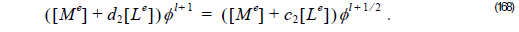Using recurrence formula Equation 118 for Padé(2,2) we get: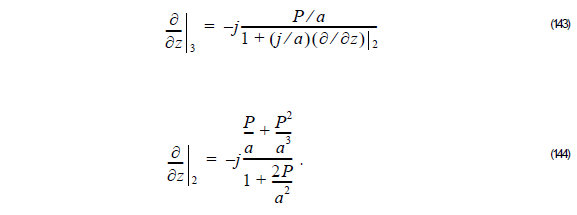Here  a = 2k0nref .

Using Equation 144 into Equation 143, we get:From Equation 145, we get:Thus,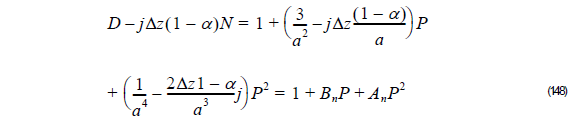and,where: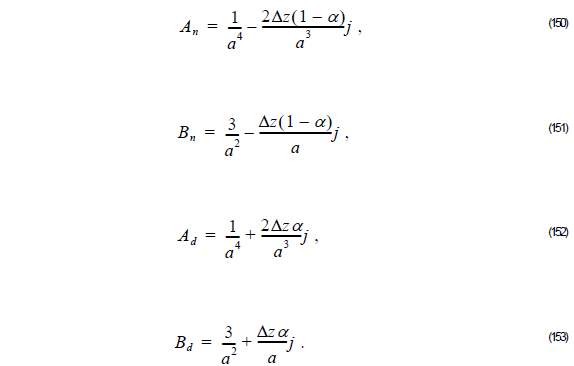Thus, the unknown field φ l + 1  at z + Δz is related to the known field φ l at z as follows:## Multistep Method

In order to solve Equation 154, we applied the multistep method developed by Hadley.

First, we rewrite Equation 154 asThen, defining the field φ l + 1 ⁄ 2   as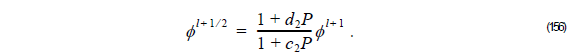We rewrite Equation 154 as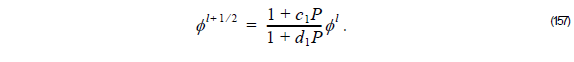Since φ l  is known, we can obtain φ l + 1 ⁄ 2  by solving: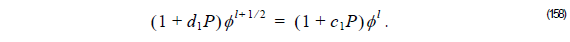If we consider FEM, we get,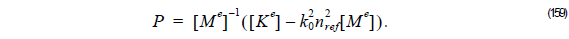Thus, we can rewrite Equation 158 as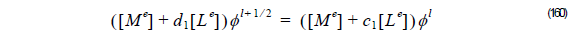here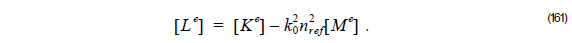Using φ l + 1 ⁄ 2 , we rewrite Equation 156 as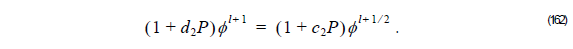If we are using FEM, we get,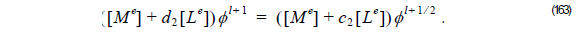Solving Equation 162 or Equation 162, we can obtain the unknown field φ l + 1 . It is apparent from the form of Equation 154 that an n th-order Padé propagator may be decomposed into an n -step algorithm for which the i th partial step takes the formwhen = 1, 2, …, n .

The run time for an n th-order propagator is obviously n times the paraxial run time. Therefore, for Padé (2,2) we follow the steps:

1          Compute φ l + 1 ⁄ 2   considering the linear system: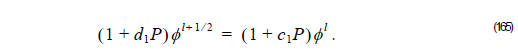If we are using FEM we get: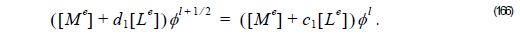2          Finally, to get the field φ l + 1 at z + Δwe solve: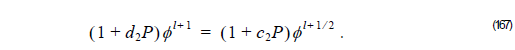If we are using FEM, we get,